# Arzelà variation

(diff) ← Older revision | Latest revision (diff) | Newer revision → (diff)
A numerical characteristic of a function of several variables, which may be regarded as the multi-dimensional analogue of the variation of a function in one unknown. Let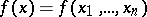be a real-valued function given on an-dimensional parallelepipedon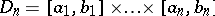,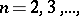and let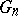be the class of all continuous vector functions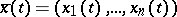,, such that each of the functions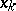is non-decreasing on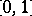, and with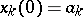,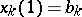,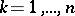. Then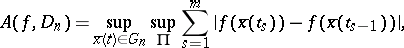where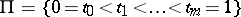is an arbitrary system of points in. This definition for the casewas proposed by C. Arzelà  (see also , p. 543). If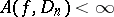, one says thathas bounded (finite) Arzelà variation on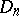, and the class of all such functions is denoted by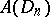. For a function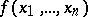to belong to the classit is necessary and sufficient that there exists a decomposition, where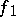andare finite non-decreasing functions on. A functionis called non-decreasing onif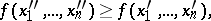for(). The classcontains the class of functions of bounded Hardy variation on.